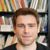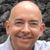## Julia Community 🟣

Arturo Erdély

Posted on • Updated on

# Dictionaries are onto functions

Dictionaries in Julia (and other programming languages) are onto functions, mathematically speaking. That is to say

$f:A\rightarrow B$
where $f$ is a function defined for every $x\in A,$ and also for every $y\in B$ there exists at least one $x\in A$ such that $f(x)=y,$ that is an onto (or surjective) function. The inverse image of $f$ is a function
$f^{(-1)}:\wp(B)\rightarrow\wp(A)$
where $\wp(B)$ is the collection of all the subsets of $B,$ that is the power set of $B,$ and where
$f^{(-1)}(C) = \{x \in A: f(x) \in C\}$
for any $C \subseteq B.$ In other words, if $f$ is a function that maps the elements of a set $A$ onto a set $B$ and if $C$ is a subset of $B$ then the inverse image $f^{(-1)}(C)$ is a subset of $A$ with all the elements that are mapped by $f$ onto the set $C.$

If D is a Dict then D is the equivalent to $f$ where $A =$ keys(D) and $B =$ values(D). To define the inverse image function $f^{(-1)}$ it is just necesary to code:

invImg(C::Set, D::Dict) = Set(filter(x -> D[x] ∈ C, keys(D)))


For example:

D = Dict([1,2,3,4,5,6,7] .=> ['a','a','b','a','b','a','c'])
C = Set(['a','c'])
invImg(C, D)


obtaining $f^{(-1)}(C) = \{1,2,4,6,7\}$ as expected.